# Hypothesis Testing

Hypothesis testing helps an organization determine whether making a change to a process input (x) significantly changes the output (y) of the process. It statistically determine if there are differences between two or more process outputs. Hypothesis testing is used to help determine if the variation between groups of data is due to true differences between the groups or is the result of common cause variation, which is the natural variation in a process.

This tool is most commonly used in the Analyze step of the DMAIC method to determine if different levels of a discrete process setting (x) result in significant differences in the output (y). An example would be “Do different regions of the country have different defect levels?” This tool is also used in the Improve step of the DMAIC method to prove a statistically significant difference in “before” and “after” data. It Identifies whether a particular discrete x has an effect on the y. This also checks for the statistical significance of differences. In other words, it helps determine if the difference observed between groups is bigger than what you would expect from common-cause variation alone. This gives  a p-value, which is the probability that a difference you observe is as big as it is only because of common-cause variation. It can be  used to compare two or more groups of data, such as “before” and “after” data.

Hypothesis testing assists in using sample data to make decisions about population parameters such as averages, standard deviations, and proportions. Testing a hypothesis using statistical methods is equivalent to making an educated guess based on the probabilities associated with being correct. When an organization makes a decision based on a statistical test of a hypothesis, it can never know for sure whether the decision is right or wrong, because of sampling variation. Regardless how many times the same population is sampled, it will never result in the same sample mean, sample standard deviation, or sample proportion. The real question is whether the differences observed are the result of changes in the population, or the result of sampling variation. Statistical tests are used because they have been designed to minimize the number of times an organization can make the wrong decision. There are two basic types of errors that can be made in a statistical test of a hypothesis:

1. A conclusion that the population has changed when in fact it has not.
2. A conclusion that the population has not changed when in fact it has.

The first error is referred to as a type I error. The second error is referred to as a type II error. The probability associated with making a type I error is called alpha (α) or the α risk. The probability of making a type II error is called beta (β) or the β risk. If the α risk is 0.05, any determination from a statistical test that the population has changed runs a 5% risk that it really has not changed. There is a 1 – α, or 0.95, confidence that the right decision was made in stating that the population has changed. If the β risk is 0.10, any determination from a statistical test that there is no change in the population runs a 10% risk that there really may have been a change. There would be a 1 – β, or 0.90, “power of the test,” which is the ability of the test to detect a change in the population. A 5% α risk and a 10% β risk are typical thresholds for the risk one should be willing to take when making decisions utilizing statistical tests. Based upon the consequence of making a wrong decision, it is up to the Black Belt to determine the risk he or she wants to establish for any given test, in particular the α risk. β risk, on the other hand, is usually determined by the following:

• δ: The difference the organization wants to detect between the two population parameters. Holding all other factors constant, as the δ increases, the β decreases.
• σ: The average (pooled) standard deviation of the two populations. Holding all other factors constant, as the σ decreases, the β decreases.
• n: The number of samples in each data set. Holding all other factors constant, as the n increases, the β decreases.
• α: The alpha risk or decision criteria. Holding all other factors constant, as the α decreases, the β increases.

Most statistical software packages will have programs that help determine the proper sample size, n, to detect a specific δ, given a certain σ and defined α and β risks.

#### p-Value

How does an organization know if a new population parameter is different from an old population parameter? Conceptually, all hypothesis tests are the same in that a signal (δ)-to-noise (σ) ratio is calculated (δ/σ) based on the before and after data. This ratio is converted into a probability, called the p-value, which is compared to the decision criteria, the α risk. Comparing the p-value (which is the actual α of the test) to the decision criteria (the stated α risk) will help determine whether to state the system has or has not changed.
Unfortunately, a decision in a hypothesis can never conclusively be defined as a correct decision. All the hypothesis test can do is minimize the risk of making a wrong decision. Conducting a hypothesis test is analogous to a prosecuting attorney trying a case in a court of law. The objective of the prosecuting attorney is to collect and present enough evidence to prove beyond a reasonable doubt that a defendant is guilty. If the attorney has not done so, then the jury will assume that not enough evidence has been presented to prove guilt; therefore, they will conclude the defendant is not guilty. If one want to wants to make a change to an input (x) in an existing process to determine a specified improvement in the output (y), he or she will need to collect data after the change in x to demonstrate beyond some criteria (the α risk) that the specified improvement in y was achieved.

#### The following steps describe how to conduct a hypothesis test

1.  Define the problem or issue to be studied.
2.  Define the objective.
3. State the null hypothesis, identified as H0.
The null hypothesis is a statement of no difference between the before and after states (similar to a defendant being not guilty in court).
H0: μbefore = μafter
The goal of the test is to either reject or not reject H0.
4. State the alternative hypothesis, identified as Ha.
• The alternative hypothesis is what one is trying to prove and can be one of the following:
• Ha: μbefore    μafter (a two-sided test)
• Ha: μbefore < μafter (a one-sided test)
• Ha: μbefore > μafter (a one-sided test)
• The alternative chosen depends on what one is trying to prove. In a two-sided test, it is important to detect differences from the hypothesized mean, μbefore, that lie on either side of μbefore. The α risk in a two-sided test is split on both sides of the histogram. In a one-sided test, it is only important to detect a difference on one side or the other.
5. Determine the practical difference (δ).
The practical difference is the meaningful difference the hypothesis test should detect.
6. Establish the α and β risks for the test.
7. Determine the number of samples needed to obtain the desired β risk. Remember that the power of the test is (1-β).
8. Collect the samples and conduct the test to determine a p-value.
Use a software package to analyze the data and determine a p-value.
9. Compare the p-value to the decision criteria (α risk) and determine whether to reject H0 in favor of Ha, or not to reject H0.
• If the p-value is less than the α risk, then reject H0 in favor Ha.
• If the p-value is greater than the α risk, there is not enough evidence to reject H0.

Depending on the population parameter of interest there are different types of hypothesis tests; these types are described in the following table.The table is divided into two sections: parametric and non-parametric. Parametric tests are used when the underlying distribution of the data is known or can be assumed (e.g., the data used for t-testing should subscribe to the normal distribution). Non-parametric tests are used when there is no assumption of a specific underlying distribution of the data.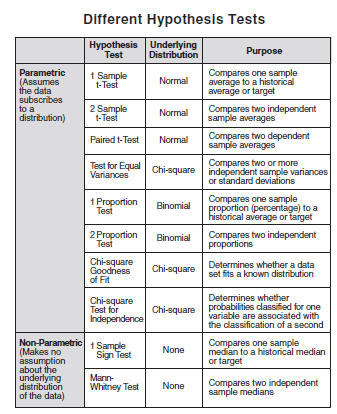A number of commonly used hypothesis test terms are presented below.

1. #### Null Hypothesis

This is the hypothesis to be tested. The null hypothesis directly stems from the problem statement and is denoted as H0. Examples:-

• If one is investigating whether a modified seed will result in a different yield/acre, the null hypothesis (two-tail) would assume the yields to be the same H0: Ya = Yb.
•  If a strong claim is made that the average of process A is greater than the average of process B, the null hypothesis (one-tail) would state that process A ≤ process B. This is written as H0: A ≤ B.

The procedure employed in testing a hypothesis is strikingly similar to a court trial. The hypothesis is that the defendant is presumed not guilty until proven guilty. However, the term innocent does not apply to a null hypothesis. A null hypothesis can only be rejected, or fail to be rejected, it cannot be accepted because of a lack of evidence to reject it. If the means of two populations are different, the null hypothesis of equality can be rejected if enough data is collected. When rejecting the null hypothesis, the alternate hypothesis must be accepted.

2. #### Test Statistic

In order to test a null hypothesis, a test calculation must be made from sample information. This calculated value is called a test statistic and is compared to an appropriate critical value. A decision can then be made to reject or not reject the null hypothesis.

3. #### Types of Errors

When formulating a conclusion regarding a population based on observations from a small sample, two types of errors are possible:

• Type I error: This error occurs when the null hypothesis is rejected when it is, in fact, true. The probability of making a type I error is called α (alpha) and is commonly referred to as the producer’s risk (in sampling). Examples are:
incoming products are good but called bad; a process change is thought to be different when, in fact, there is no difference.
• Type II error: This error occurs when the null hypothesis is not rejected when it should be rejected. This error is called the consumer’s risk (in sampling) , and is denoted by the symbol β (beta). Examples are: incoming products are bad, but called good; an adverse process change has occurred but is thought to be no different.

The degree of risk (α) is normally chosen by the concerned parties (α is normally taken as 5%) in arriving at the critical value of the test statistic. The assumption is  that a small value for α is desirable. Unfortunately, a small α risk increases the β risk. For a fixed sample size, α and β are inversely related. Increasing the sample size can reduce both the α and β risks.Any test of hypothesis has a risk associated with it and one is generally concerned with the or risk (a type I error which rejects the null hypothesis when it is true). The level of this α risk determines the level of confidence (1 – α) that one has in the conclusion. This risk factor is used to determine the critical value of the test statistic which is compared to a calculated value.

4. #### One-Tail Test

If a null hypothesis is established to test whether a sample value is smaller or larger than a population value, then the entire or risk is placed on one end of a distribution curve. This constitutes a one-tail test.

• A study was conducted to determine if the mean battery life produced by a new method is greater than the present battery life of 35 hours. In this case, the entire or risk will be placed on the right tail of the existing life distribution curve.
H0: new< or = to present                   H1: new>present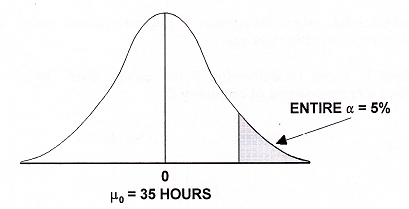Determine if the true mean is within the or critical region.
• A chemist is studying the vitamin levels in a brand of cereal to determine if the process level has fallen below 20% of the minimum daily requirement. It is the manufacturer’s intent to never average below the 20% level. A one-tail test would be applied in this case, with the entire at risk on the left tail.
H0: level > or = 20%                   H1: level < 20%Determine if the true mean is within the α  critical region.
5. #### Two-Tail Test

If a null hypothesis is established to test whether a population shift has occurred, in either direction, then a two-tail test is required. The allowable α error is generally divided into two equal parts. Examples:

• An economist must determine if unemployment levels have changed significantly over the past year.
• A study is made to determine if the salary levels of company A differ significantly from those of company B.

H0: levels are =                                                     H1: levels are ≠Determine if the true mean is within either the upper or lower α critical regions.

6. #### Practical Significance vs. Statistical Significance

The hypothesis is tested to determine if a claim has significant statistical merit. Traditionally, levels of 5% or 1% are used for the critical significance values. If the calculated test statistic has a p-value below the critical level then it is deemed to be statistically significant. More stringent critical values may be required when human injury or catastrophic loss is involved. Less stringent critical values may be advantageous when there are no such risks and the potential economic gain is high. On occasion, an issue of practical versus statistical significance may arise. That is, some hypothesis or claim is found to be statistically significant, but may not be worth the effort or expense to implement. This could occur if a large sample was tested to a certain value, such as a diet that results in a net loss of 0.5 pounds for 10,000 people. The result is statistically significant, but a diet losing 0.5 pounds per person would not have any practical significance. The  issues of practical significance will often occur if the sample size is not adequate. A power analysis may be needed to aid in the decision- making process.

7. #### Power of Test H0 : μ = μ0

Consider a null hypothesis that a population is believed to have mean μ0= 70.0 and σx = 0.80. The 95% confidence limits are 70±(1.96)(0.8) = 71.57 and 68.43. One accepts the hypothesis μ = 70 if (X-bar)s are between these limits. The alpha risk is that  sample means will exceed those limits. One can ask “what if” questions such as, “What if” μ shifts to 71, would it be detected?” There is a risk that the null hypothesis would be accepted even if the shift occurred. This risk is termed β. The value of β is large if μ is close to μ0 and small if μ is very different from μ0. This indicates that slight differences from the hypothesis will be difficult to detect and large differences will be easier to detect. The normal distribution curves below show the null and alternative hypotheses. If the process shifts from 70 to 71, there is a 76% probability that it would not be detected.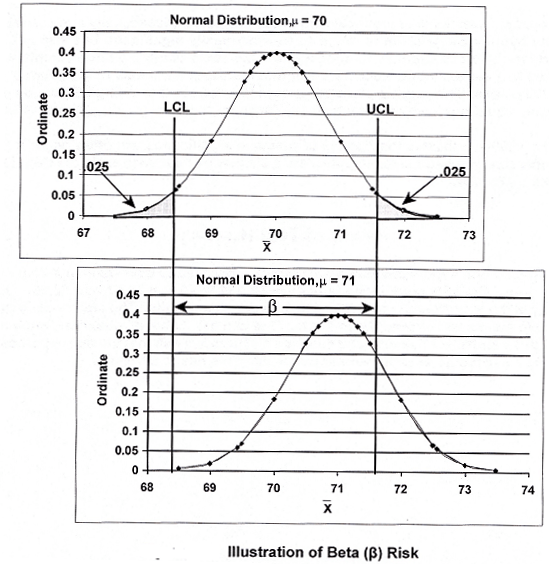To construct a power curve, 1 – β is plotted against alternative values of μ. The power curve for the process under discussion is shown below. A shift in a mean away from the null increases the probability of detection. In general, as alpha increases, beta decreases and the power of 1 – β increases. One can say that a gain in power can be obtained by accepting a lower level of protection from the alpha error. Increasing the sample size makes it possible to decrease both alpha and beta and increase power.The concept of power also relates to experimental design and analysis of variance.
The following equation briefly states the relationship for ANOVA.
1 – β = P(Reject H0 /H0 is false)
1 – β = Probability of rejecting the null hypothesis given that the null hypothesis is false.

8. #### Sample Size

In the statistical inference discussion thus far, it has been assumed that the sample size (n) for hypothesis testing has been given and that the critical value of the test statistic will be determined based on the α error that can be tolerated. The ideal procedure, however, is to determine the α and β error desired and then to calculate the sample size necessary to obtain the desired decision confidence.

The sample size (n) needed for hypothesis testing depends on:

• The desired type I (α) and type II (β) risk
• The minimum value to be detected between the population means (μ – μ0)
• The variation in the characteristic being measured (S or σ)

Variable data sample size, only using a, is illustrated by the following: Assume in a pilot process one wishes to determine whether an operational adjustment will alter the process hourly mean yield by as much as 4 tons per hour. What is the minimum sample size which, at the 95% confidence level (Z=1.96), would confirm the significance of a mean shift greater than 4 tons per If hour? Historic information suggests that the standard deviation of the hourly output  is 20 tons. The general sample size equation for variable data (normal distribution) is: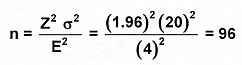Obtain 96 pilot hourly yield values and determine the hourly average. If this mean deviates by more than 4 tons from the previous hourly average, a significant change at the 95% confidence level has occurred. If the sample mean deviates by less than 4 tons/hr, the observable mean shift can be explained by chance cause.

For binomial data, use the following formula: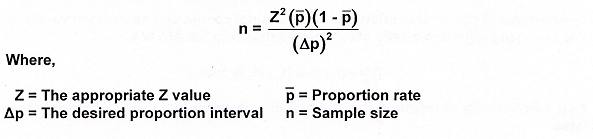9. #### Estimators

In analyzing sample values to arrive at population probabilities, two major estimators are used: point estimation and interval estimation. For example, Consider the following tensile strength readings from 4 piano wire segments: 28.7, 27.9, 29.2 and 26.5 psi. Based on this data, the following expressions are true:

1. Point estimation: If a single estimate value is desired (i.e., the sample average), then a point estimate can be obtained.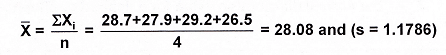28.08 psi is the point estimate for the population mean.
2. Interval Estimate or Cl (Confidence Interval): From sample data one can calculate the interval within which the population mean is predicted to fall. A Confidence intervals are always estimated for population parameters and, in general, are derived from the mean and standard deviation of sample data. For small samples, a critical value from the t distribution is required and for 95% confidence, t = 3.182 for n-1 degrees of freedom. The CI equation and interval would be: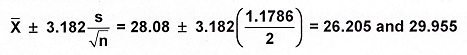If the population sigma is known (say σ = 2 psi), the Z distribution is used. The critical Z value for 95% conﬁdence is 1.96. The CI equation and interval would be: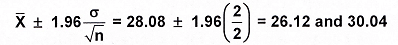A confidence interval is a two-tail event and requires critical values based on an alpha/2 risk in each tail.  Other confidence interval formulas exist. These include percent nonconforming, Poisson distribution data and very small sample size data.
1. ### Confidence Intervals for the Mean

1. #### Continuous Data – Large Samples

Use the normal distribution to calculate the confidence interval for the mean.Example: The average of 100 samples is 18 with a population standard deviation of 6. Calculate the 95% confidence interval for the population mean.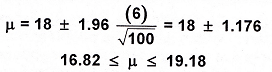2. ##### Continuous Data – Small Samples

If a relatively small sample is used (<30) then the t distribution must be used.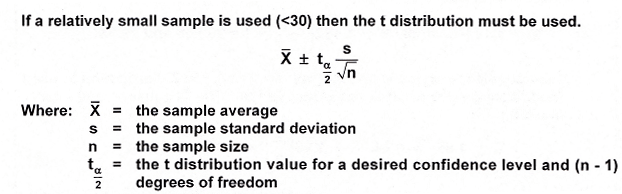Example : Use the same values as in the prior example except that the sample size is 25.2. #### Confidence Intervals for Variation

The confidence intervals for the mean were symmetrical about the average. This is  not true for the variance, since it is based on the chi square distribution. The formula is :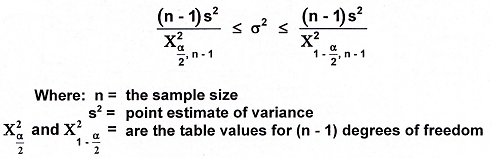Example: The sample variance for a set of 25 samples was found to be 36. Calculate the 90% confidence interval for the population variance.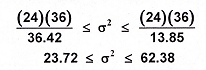3. #### Confidence Intervals for Proportion

For large sample sizes, with n(p) and n(1-p) greater than or equal to 4 or 5, the normal distribution can be used to calculate a confidence interval for proportion. The following formula is used: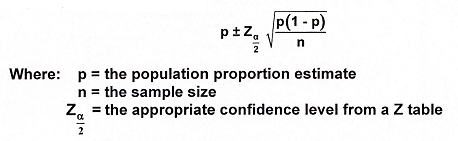Example: If 16 defectives were found in a sample size of 200 units, calculate the 90% confidence interval for the proportion.### Hypotheses Tests for Comparing  Single Population

We begin by considering hypothesis tests to compare parameters of a single population, such as , and fraction defective p, to specified values. For example, viscosity may be an important characteristic in a process validation experiment and we may want to determine if the population standard deviation of viscosity is less than a certain value or not. Additional examples of such comparisons are suggested by the following questions.

1. Is the process centered on target? Is the measurement bias acceptable?
2. Is the measurement standard deviation less than 5% of the specification width? Is the process standard deviation less than 10% of the specification width?
3. Let p denote the proportion of objects in a population that possess a certain property such as products that exceed a certain hardness, or cars that are domestically manufactured. Is this proportion p greater than a certain specified value?

1. #### Z Test

When the population follows a normal distribution and the population standard deviation, σx, is known, then the hypothesis tests for comparing a population mean, μ, with a fixed value, μ0, are given by the following:

• H0: μ = μ0                  H1: μ ≠ μ0
• H0: μ ≤ μ0                  H1: μ> μ0
• H0: μ ≥ μ0                  H1: μ < μ0

The null hypothesis is denoted by H0 and the alternative hypothesis is denoted by H1. The test statistic is given by: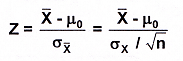where the sample average is X-bar, the number of samples is n and the standard  deviation of the mean is σx. Note, if n > 30, that the sample standard deviation, s, is  often used as an estimate of the population standard deviation, σx. The test statistic, Z, is compared with a critical value Zα  or Zα/2, which is based on a significance level,α, for a one—tailed test or α/2 for a two-tailed test. If the H1 sign is ≠, it is a two-tailed test. If the H1 sign is >, it is a right, one-tailed test, and if the H1 sign is <, it is a left, one-tailed test.
Example: The average vial height from an injection molding process has been 5.00″ with a standard deviation of 0.12″. An experiment is conducted using new material which yielded the following vial heights: 5.10″, 4.90″, 4.92″, 4.87″, 5.09″, 4.89″, 4.95″, and 4.88″. Can one state with 95% confidence that the new material is producing shorter vials with the existing molding machine setup? This question involves an inference about a population mean with a known sigma. The Z test applies. The null and alternative hypotheses are:

H0: μ ≥ μ0                  H1: μ < μ0

H0: μ ≥ 5.00″                  H1: μ <5.00″

The sample average is (X-bar) = 4.95″ with n = 8 and the population standard deviation is σx = 0.12″. The test statistic is: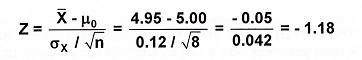Since the H, sign is <, it is a left, one-tailed test and with a 95% confidence, the level of significance, α = 1 – 0.95 = 0.05. Looking up the critical value in a normal distribution or Z table, one finds Z0.05 = -1.645. Since the test statistic, -1 .18, does not fall in the reject (or critical) region, the null hypothesis cannot be rejected. There is insufficient evidence to conclude that the vials made with the new material are shorter.
If the test statistic had been, for example -1.85, we would have rejected the null hypothesis and concluded the vials made with the new material are shorter.2. #### Student’s t Test

This technique was developed by W. S. Gosset and published in 1908 under the pen name “Student.” Gosset referred to the quantity under study as t. The test has since been known as the student’s t test. The student’s t distribution applies to samples drawn from a normally distributed population. It is used for making inferences about a population mean when the population variance, σ2, is unknown and the sample size, n, is small. The use of the t distribution is never wrong for any sample size. However, a sample size of 30 is normally the crossover point between the t and Z tests. The test statistic formula is: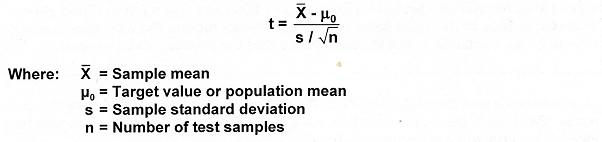The null and alternative hypotheses are the same as were given for the Z test. The test statistic, t, is compared with a critical value, tα  or tα/2, which is based on a significance level,α, for a one—tailed test or α/2 for a two-tailed test and the number of degrees of freedom, d.f. The degrees of freedom is determined by the number of samples, n, and is simply: dt=n-1

Example: The average daily yield of a chemical process has been 880 tons (μ = 880 tons). A new process has been evaluated for 25 days (n = 25) with a yield of 900 tons (X-bar) and sample standard deviation, s = 20 tons. Can one say with 95% confidence that the process has changed?

The null and alternative hypotheses are:

H0: μ = μ0                  H1: μ ≠ μ0

H0: μ = 880 tons                  H1: μ ≠ 880 tons

The test statistic calculation is:

Since the H1 sign is ≠, it is a two-tailed test and with a 95% confidence, the level of significance, α = 1 – 0.95 = 0.05. Since it is a two-tail test, α/2 is used to determine the critical values. The degrees of freedom d.f. = n – 1 = 24. Looking up the critical values in a t distribution table, one finds t0.025.= -2.064 and t0.975 = 2.064. Since the test statistic, 5, falls in the right-hand reject (or critical) region, the null hypothesis is rejected. We conclude with 95% confidence that the process has changed.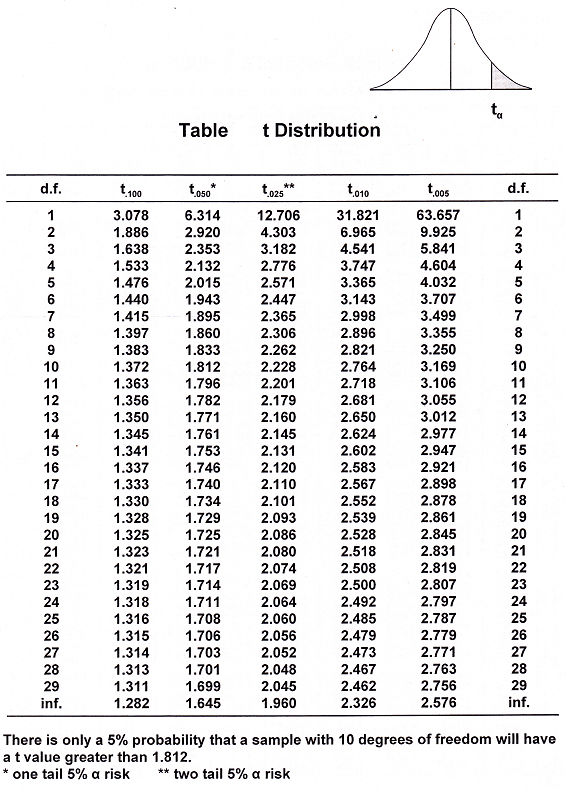One underlying assumption is that the sampled population has a normal probability distribution. This is a restrictive assumption since the distribution of the sample is unknown. The t distribution works well for distributions that are bell-shaped.

#### Chi Square (χ2) Test

Standard deviation (or variance) is fundamental in making inferences regarding the population mean. In many practical situations, variance (σ2) assumes a position of greater importance than the population mean. Consider the following examples:

1. A shoe manufacturer wishes to develop a new sole material with a more stable wear pattern. The wear variation in the new material must be smaller than the variation in the existing material.
2. An aircraft altimeter manufacturer wishes to compare the measurement precision among several instruments.
3. Several inspectors examine finished parts at the end of a manufacturing process. Even when the same lots are examined by different inspectors, the number of defectives varies. Their supervisor wants to know if there is a significant difference in the knowledge or abilities of the inspectors.

The above problems represent a comparison of a target or population variance with an observed sample variance, a comparison between several sample variances, or a comparison between frequency proportions. The standardized test statistic is called the Chi Square (χ2)test. Population variances are distributed according to the chi square distribution. Therefore, inferences about a single population variance will be based on chi square. The chi square test is widely used in two applications.
Case I. Comparing variances when the variance of the population is known.
Case ll. Comparing observed and expected frequencies of test outcomes when there is no defined population variance (attribute data).
When the population follows a normal distribution, the hypothesis tests for comparing a population variance, 0:, with a fixed value, 0:, are given by the following:

• H0: σx2 = σ02                  H1: σx2 ≠ σ02
• H0: σx2 ≤ σ02                  H1: σx2> σ02
• H0: σx2 ≥ σ02                  H1x2 < σ02

The null hypothesis is denoted by H0 and the alternative hypothesis is denoted by H1. The test statistic is given by: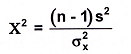Where the number of samples is n and the sample variance is s2. The test statistic, A χ2, is compared with a critical value χα2, or χα/22, which is based on a significance level, α, for a one-tailed test or α/2 for a two-tailed test and the number of degrees of freedom, d.f. The degrees of freedom is determined by the number of samples, n, and is simply:  d,f.=n-1

If the H1 sign is≠, it is a two-tailed test. If the H1 sign is >, it is a right, one-tailed test, and if the H1 sign is <, it is a left, one-tailed test.

The χ2 distribution looks like so:Please note, unlike the Z and t distributions, the tails of the chi square distribution are non-symmetrical.

• Chi square Case I. Comparing Variances When the Variance of the Population Is Known.

Example: The R & D department of a steel plant has tried to develop a new steel alloy with less tensile variability. The R & D department claims that the new material will show a four sigma tensile variation less than or equal to 60 psi 95% of the time. An eight sample test yielded a standard deviation of 8 psi. Can a reduction in tensile
strength variation be validated with 95% confidence?

Solution: The best range of variation expected is 60 psi. This translates to a sigma of 15 psi (an approximate 4 sigma spread covering 95.44% of occurrences).

H0: σx2 ≥ σ02                  H1x2 < σ02

H0: σx2 ≥ 152                  H1x2 < 152

From the chi square table: Because S is less than σ, this is a left tail test with n – 1 = 7. The critical value for 95% confidence is 2.17. That is, the calculated value will be less than 2.17, 5% of the time. Please note that if one were looking for more variability in the process a right tail rejection region would have been selected and the critical value would be 14.07.
The calculated statistic is:=(7)(8)2/(15)2=1.99
Since 1.99 is less than 2.17, the null hypothesis must be rejected. The decreased variation in the new steel alloy tensile strength supports the R & D claim.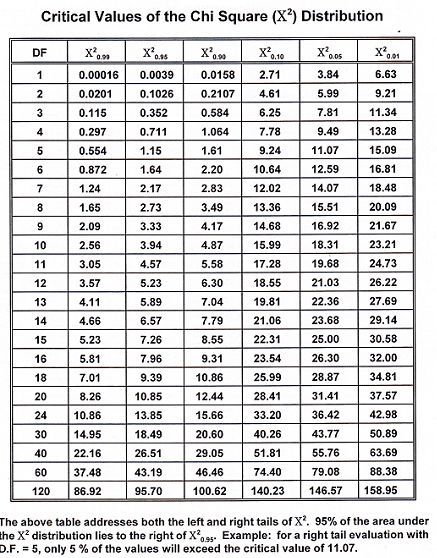• #### Chi square Case ll. Comparing Observed and Expected Frequencies of Test Outcomes. (Attribute Data)

It is often necessary to compare proportions representing various process conditions. Machines may be compared as to their ability to produce precise parts. The ability of inspectors to identify defective products can be evaluated. This application of chi square is called the contingency table or row and column analysis.
The procedure is as follows:

1. Take one subgroup from each of the various processes and determine the  observed frequencies (0) for the various conditions being compared.
2. Calculate for each condition the expected frequencies (E) under the assumption that no differences exist among the processes.
3.  Compare the observed and expected frequencies to obtain “reality.” The following calculation is made for each condition:4. Total all the process conditions:5. A critical value is determined using the chi square table with the entire level of significance, σ, in the one-tail, right side, of the distribution. The degrees of freedom is determined from the calculation (R-1)(C-1) [the number of rows minus 1 times the number of columns minus 1 ].
6. A comparison between the test statistic and the critical value confirms if a ) significant difference exists (at a selected confidence level).

Example: An airport authority wanted to evaluate the ability of three X-ray inspectors to detect key items. A test was devised whereby transistor radios were placed in ninety pieces of luggage. Each inspector was exposed to exactly thirty of the pre selected and “bugged” items in a random fashion. The observed results are summarized below.Is there any significant difference in the abilities of the inspectors? (95%  conﬁdence)
Null hypothesis:
There is no difference among  three inspectors, H0: p1 = p2 = p3
Alternative hypothesis:
At least one of the proportions is different, H1: p1 ≠ p2 ≠ p3
The degrees of freedom = (rows – 1)(columns – 1) = (2-1)(3-1) = 2
The critical value of χ2 for DF = 2 and d = 0.05 in the one-tail, right side of the distribution, is 5.99 . There is only a 5% chance that the calculated value of χ2 will exceed 5.99.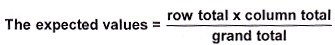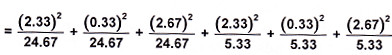= 0.220 + 0.004 + 0.289 + 1.019 + 0.020 + 1.333
χ2 = 2.89

Since the calculated value of χ2 is less than the previously calculated critical value of 5.99 and this is a right tail test, the null hypothesis cannot be rejected. There is insufficient evidence to say with 95% confidence that the abilities of the inspectors differ.

#### p Test

When testing a claim about a population proportion, with a fixed number of independent trials having constant probabilities, and each trial has two outcome possibilities (a binomial experiment), a p test can be used. When np < 5 or n(1-p) < 5, the binomial distribution is used to test hypotheses relating to proportion.
If conditions that np ≠ 5 and n(1-p)≠5 are met, then the binomial distribution of sample proportions can be approximated by a normal distribution. The hypothesis tests for comparing a sample proportion, p, with a fixed value, po, are given by the following:

• H0: p = p0                  H1: p ≠ p0
• H0: p ≤ p0                  H1: p> p0
• H0: p ≥ μ0                  H1: μ < p0

The null hypothesis is denoted by H0 and the alternative hypothesis is denoted by H1. The test statistic is given by: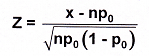Where the number of successes is x and the number of samples is n. The test statistic, Z, is compared with a critical value Zα  or Zα/2, which is based on a significance level,α, for a one—tailed test or α/2 for a two-tailed test .If the H1 sign is >, it is a right, one-tailed test, and if the H1 sign is <, it is a left, one-tailed test.

Example. A local newspaper stated that less than 10% of the rental properties did not allow renters with children. The city council conducted a random sample of 100 units and found 13 units that excluded children. Is the newspaper statement wrong based upon this data? In this case H0: p ≤ 0.1 and H1: p> 0.1 In this case p0 = 0.1 and the computed Z value is

Z=For α= 0.05, Z = 1.64 and the newspaper statement cannot be rejected based upon this data at the 95% level of confidence.

### Hypotheses Test for Comparing  two Population

Here we considers hypothesis tests to compare parameters of two populations with each other. For example, we may want to know if after a process change the process is different from the way it was before the change. The data after the change constitute one population to be compared with the data prior to change, which constitute the other population. Some specific comparative questions are: Has the process mean changed? Has the process variability reduced? If the collected data are discrete, such as defectives and non defectives, has percent defective changed?

#### Z Test.

The following test applies when we want to compare two population
means and the variance of each population is either known or the sample size is large (n > 30). Let denote the population mean, sample size, sample average and population standard deviation for the first population and let  represent the same quantities for the second population. The hypotheses being compared are H0: μ1 = μ2  and             H1: μ1 ≠μ2.  Under the null hypothesis

Therefore, the test statistic Z =

has a standard normal distribution. If the computed value of Z exceeds the critical value, the null hypothesis is rejected.

Example. We want to determine whether the tensile strength of products from two suppliers are the same. Thirty samples were tested from each supplier with the following results:  and Z =

The Z value for  α= 0.001 is 3.27; hence, the two means are different
with 99.9% confidence.

#### Independent t-Test.

This test is used to compare two population means when the sample sizes are small, the population variances are unknown but may be assumed to be equal. In this situation, a pooled estimate of the standard deviation is used to conduct the t-test. Prior to using this test, it is necessary to demonstrate that the two variances are not different, which can be done by using the F-test   The hypotheses being tested are The hypotheses being compared are H0: μ1 = μ2  and H1: μ1 ≠μ2. . A pooled estimate of variance is obtained by weighting the two variances in proportion to their degrees of freedom as follows: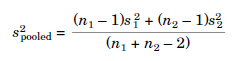The test statistic t has has a tn1+n2–2 distribution. If the computed value of t exceeds the critical value, H0 is rejected and the difference is said to be statistically significant.

Example. The following results were obtained in comparing surface soil pH at two different locations: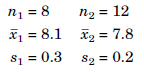Do the two locations have the same pH?
Assuming that the two variances are equal, we first obtain a pooled
estimate of variance:Spooled = 0.24. Then the t statistic is computed:For a two-sided test with = 0.05 and (n1 + n2 – 2) = 18 degrees of freedom, the critical value of t is t0.025,18 = 2.1. Since computed value of t exceeds the critical value 2.1, the hypothesis that the two locations have the same pH is rejected.

#### Independent t-Test.

This test is used to compare two population means when the sample sizes are small (n < 30), the variance is unknown, and the two population variances are not equal, which should first be demonstrated by conducting the F-test to compare two variances. The hypotheses being compared are H0: μ1 = μ2  and H1: μ1 ≠μ2. The test statistic t and the degrees of freedom ν are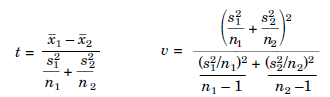If the computed t exceeds the critical t, the null hypothesis is rejected.
Example. The following data were obtained on the life of light bulbs made by two manufacturers: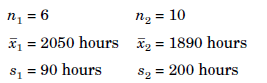Is there a difference in the mean life of light bulbs made by the two manufacturers? Assuming  that the F-test in  shows that the two standard deviations are not equal. The computed t and ν are: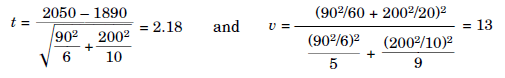For α= 0.05, tα/2,ν = 2.16. Since the computed value exceeds the critical t value, we are 95% sure that the mean life of the light bulbs from the two manufacturers is different.

#### Comparing Two Means (Paired t-test)

This test is used to compare two population means when there is a physical reason to pair the data and the two sample sizes are equal. A paired test is more sensitive in detecting differences when the population standard deviation is large. The hypotheses being compared are H0: μ1 = μ2  and H1: μ1 ≠μ2. The test statistic twhere
d = difference between each pair of values
dbar = observed mean difference
sd = standard deviation of d

Example. Two operators conducted simultaneous measurements on
percentage of ammonia in a plant gas on nine successive days to find the extent of bias in their measurements.  Since the day-to-day differences in gas composition were larger than the expected bias, the tests were designed to permit paired comparison.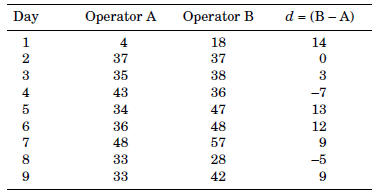For α= 0.05, t0.025,8 = 2.31. Since the computed t value is less than the critical t value, the results do not conclusively indicate that a bias exists.

#### Comparing Two Standard Deviations

##### F-Test.

This test is used to compare two standard deviations and applies for all sample sizes. The hypotheses being compared are H0: σ1 = σ2  and  H1: σ1 ≠σ2.F distribution, which is a skewed distribution and is characterized by the degrees of freedom used to estimate S1 and S2, called the numerator degrees of freedom (n1 – 1) and denominator degrees of freedom (n2 – 1), respectively. Under the null hypothesis, the F statistic becomes  S12/S22

In calculating the F ratio, the larger variance is in the numerator, so that the calculated value of F is greater than one. If the computed value of F exceeds the critical value Fα/2,n1–1,n2–1 the null hypothesis is rejected.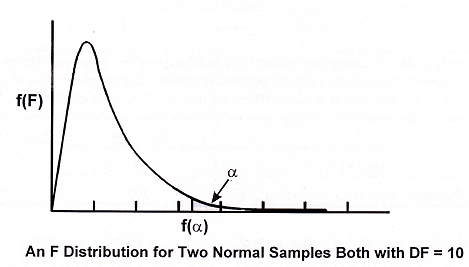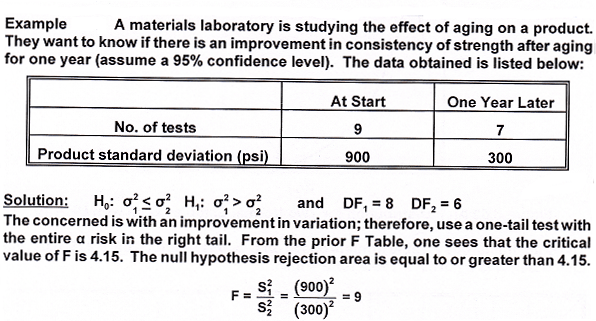Since the calculated F value is in the critical region, the null hypothesis is rejected. There is sufficient evidence to indicate a reduced variation and more consistency of strength after aging for 1 year.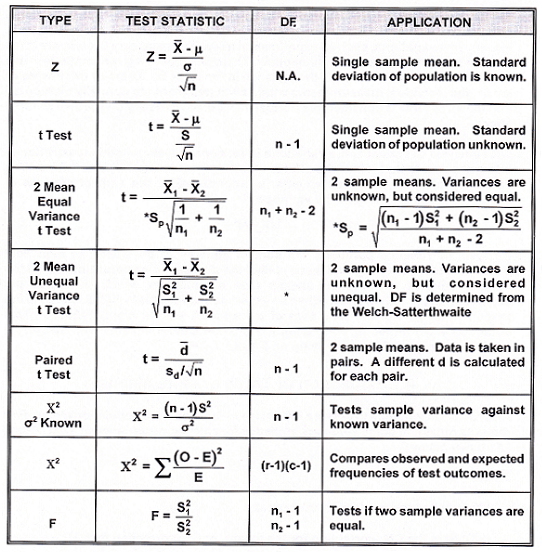## One thought on “Hypothesis Testing”

1.Mani (TGSIN - EHS) says:

Dear Pretesh Biswas San
Good morning, I am Mani from Bangalore..
I need a help from you ,Can you give few examples of efficiency and effectiveness KPIs of QMS..
Thanks & Best Regards
M.Mani